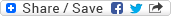## Monday, June 9, 2008

### Puzzle: 13 Red, 15 Green, and 17 Blue Chameleons

Puzzle: There are 13 Red, 15 Green, and 17 Blue Chameleons at some point of time. Whenever two Chameleons of the different colors meet both of them change their color to the third color. Is it ever possible for all Chameleons to become of the same color? Why?

Solution: Initial differences between the number of Chameleons of different colors are as follows:-

nC(r) ~ nC(g) = 2

nC(g) ~ nC(b) = 2

nC(r) ~ nC(b) = 4

Whenever two Chameleons of different colors meet, both of them convert to Chameleons of the third color i.e., the number of Chameleons of the two colors (they were before meeting) decreases by 1 whereas the number of Chameleons of the third color (they turn into after meeting) increases by 2.

Let's try to see how the difference between the number of Chameleons of different colors change once they start meeting. For example, if C(r) and C(g) meet ... then nC(r) ~ nC(g) will remain 2 only as net change will be 0. nC(g) ~ nC(b) = 2 + 1 = 3 as nC(b) increases by 2 whereas nC(g) decreases by 1 in this case. Similarly, nC(r) ~ nC(b) = 4 + 1 = 5. If we proceed like this then ultimately nC(r) will become 0 and nC(g) will remain 2, but the difference will still be 2 only. Now, we will start making C(g) and C(b) meet, but then they will start producing C(r) and ultimately nC(g) will become 0, but we'll have few nC(r) which will not be same as nC(b) and this continues... Irrespective of what combination you follow, you'll always end up being in a similar situation.

Thus, we can easily observe that the difference between Chameleons of two different colors is never going to be 0 and in absence of such a scenario we can't get all the Chameleons turning into one single color.Anonymous said...

Consider the number of chameleons modulo 3. In mod 3 arithmetic, "subtract 1" and "add 2" are exactly the same operation, so it doesn't matter which two chameleons meet at each step.
Initially R=1, G=0, B=2.
After one step R=0, G=2, B=1.
After two steps, R=2, G=1, B=0.
Then it repeats. Clearly you can never get to a position where two of the variables are 0.

Geek said...

ANonymous: Initially we have R=13, G=15, and B=17 and not what you have probably assumed (R=1, G=0, B=2).

BTW, what point you wanted to raise? Can you plz elaborate on it?Anonymous said...

I was offering another solution to the problem, but it assumes knowledge of modular arithmetic. Basically you can't achieve two zeroes because you can't escape the situation where the three numbers are an exact multiple of three, one plus a multiple of three, and two plus a multiple of three.

Geek said...

Thanks for bringing up another approach to solve the puzzle.

Pratik Poddar said...